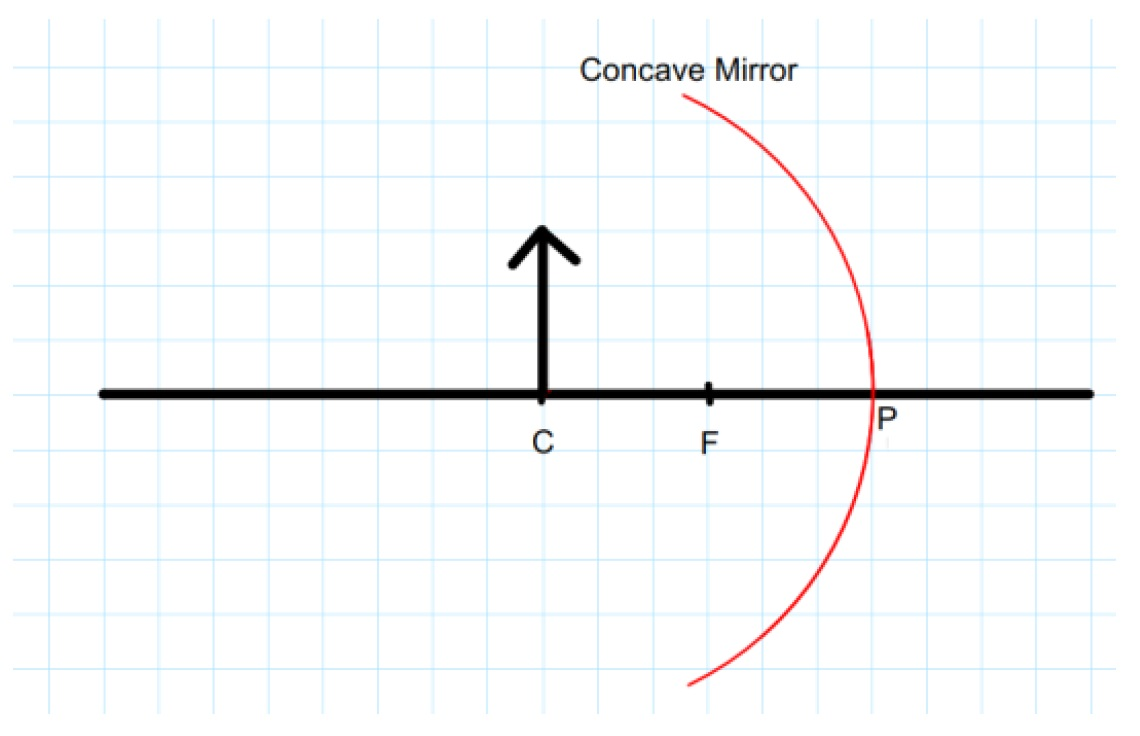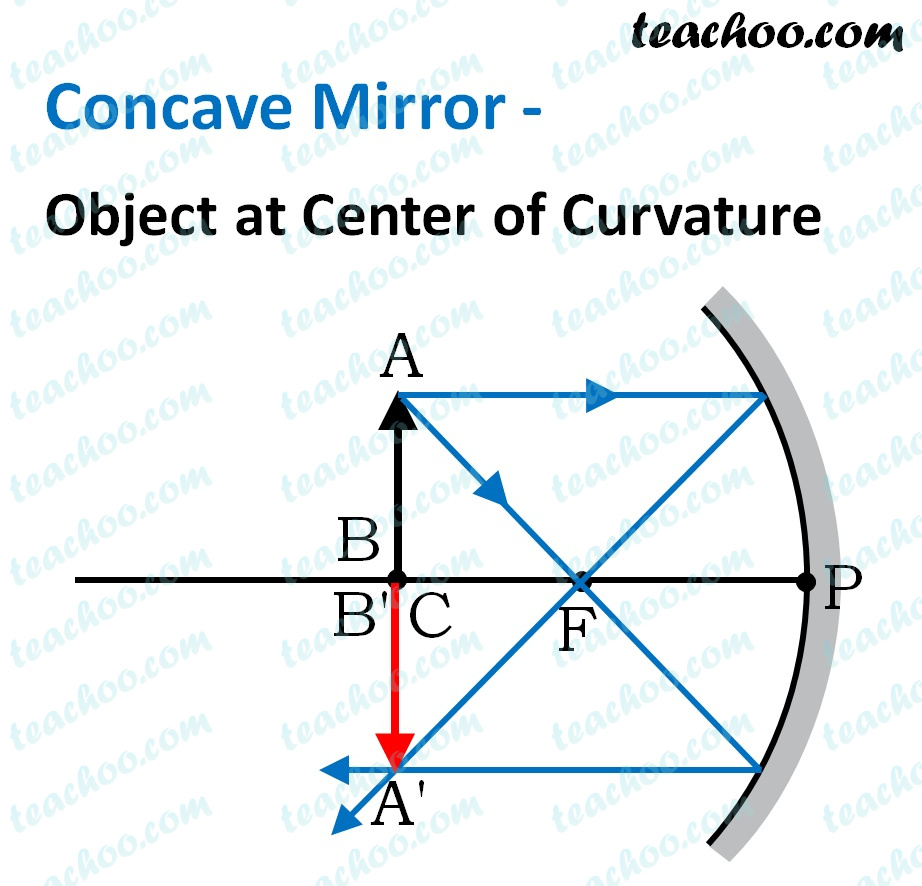Solutions - CBSE Class 10 Sample Paper for 2022 Boards - Science [MCQ]

Class 10
Solutions to CBSE Sample Paper - Science Class 10## (D) The mirror has a focal length of -6 cm and will produce an image of magnification -1.

• Since the mirror used here is a concave mirror focal length is negative
• Now, each box is of 1 cm, so,
• Focal length = -3 cm
• And since image formed is inverted and of the same size, so magnification will be -1So, the correct answer is (b)

Learn in your speed, with individual attention - Teachoo Maths 1-on-1 Class